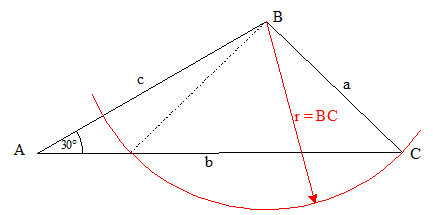# Cosine rule

## Homework Statement

ABC is a triangle
The angle A = 30 degrees
AB = 7cm
BC = 5 cm
C is an acute angle. Find the size of angle C?

## Homework Equations

I need to use the cosine rule here Cos C = (a^2 + b^2 - c^2) / 2ac

## The Attempt at a Solution

The problem is I do not know the size of side b?
So how can I attempt to resolve the answer?

Cos C = (5^2 + b^2 - 7^2) / 2*5*7

Ray Vickson
Homework Helper
Dearly Missed

## Homework Statement

ABC is a triangle
The angle A = 30 degrees
AB = 7cm
BC = 5 cm
C is an acute angle. Find the size of angle C?

## Homework Equations

I need to use the cosine rule here Cos C = (a^2 + b^2 - c^2) / 2ac

## The Attempt at a Solution

The problem is I do not know the size of side b?
So how can I attempt to resolve the answer?

Cos C = (5^2 + b^2 - 7^2) / 2*5*7

Why do you need to use the cosine rule? Did somebody tell you that you are being forced to use it?

Just use whatever tool is the most convenient. If I were doing it I would let the points be at ##A = (0,0), B = (7,0), C = (x,y).## You can use the information about angle A and distance BC to get two equations in ##x, y##. These can be reduced to a single equation in ##x##, which is solvable without too much trouble.

•SammyS
Thanks Ray but we have never used this method in class and I am sure this is the approach my teacher wants us to do it. Can I not use the cosine rule or sine rule then?

gneill
Mentor
One way or another you'll have to find side b if you're going to apply the cosine rule. If you're not comfortable jumping right into the algebra with a method you've not used before, then start with a diagram and come up with a plan from there.#### Attachments

•neilparker62
BvU
Homework Helper
I need to use the cosine rule here
Says who ?

BvU
Homework Helper
Can I not use the cosine rule or sine rule then?
What about this second option ?Ah ha!

cos A / a = sin C / c

So cos 30 / 5 = sin C / 7

So sin C = 7*(cos 30 / 5) = 1.212435565

How do I get the angle?

BvU
Homework Helper
cos A / a = sin C / c
Not how I remember the law of sinesHow do I get the angle?
The button on your calculator don't work for cosines > 1 ?Ah ha!

sin A / a = sin C / c

So sin 30 / 5 = sin C / 7

So sin C = 7*(sin 30) / 5 = 0.7

arcsin gives 44.4 degrees

Last edited:
•Delta2 and FactChecker
neilparker62
Homework Helper
In general you will use the cos rule for solving a triangle if the givens are the 3 sides or alternatively 2 sides and an angle formed between the 2 given sides.

Mark44
Mentor
Ah ha!

sin A / a = sin C / c

So sin 30 / 5 = sin C / 7

So sin C = 7*(sin 30) / 5 = 0.7

arcsin gives 44.4 degrees
It could be that there are two different triangles that satisfy the given measurements, as in the drawing in post #4. If ##\sin(C) = .7## there is also another angle that is possible for C.

Edit: Since it is stated in post #1 that C is an acute angle, the above does not apply.

Last edited:
•gneill
BvU
Homework Helper
C is an acute angle

•gneill
gneill
Mentor
It could be that there are two different triangles that satisfy the given measurements, as in the drawing in post #4. If sin(C)=.7sin⁡(C)=.7\sin(C) = .7 there is also another angle that is possible for C.
Actually, that was my primary reason for posting the diagram. Too subtle?

Mark44
Mentor
C is an acute angle
Missed that in the first post.

gneill
Mentor
Maybe too subtle, since the OP didn't seem to pick up on it.Okay, will a tad more blatant in future. I hate blatant when gently nudgingSammyS
Staff Emeritus
Homework Helper
Gold Member
Actually, that was my primary reason for posting the diagram. Too subtle?
I thought that it was just subtle enough.

symbolipoint
Homework Helper
Gold Member
I drew the diagram according to original problem description, and intuition tells me that b, and angle B, and angle C are not locked into any set of single values. Not a unique triangle. That's only first impression.

Thinking further,
forget about trying Law Of Cosines.

Law Of Sines seems fitting:

sin(C)/7=sin(30)/5

sin(C)=(7/5)sin(30)

sin(C)=7/10
and from this you could get value for angle C.

Now, you could use AB, BC, and angle at B, to find b, using Law Of Cosines.; but you could use Law Of Sines again if you want.

BvU
Homework Helper
I drew the diagram according to original problem description, ....
Why come with this now, when OP has cracked the case in #9 ?

symbolipoint
Homework Helper